# Sign of a expression

What is the sign of expression:

minus 18 start fraction 9 divided by 17 end fraction plus left parenthesis minus 18 start fraction 9 divided by 17 end fraction right parenthesis?

x =  -19.0588

### Step-by-step explanation: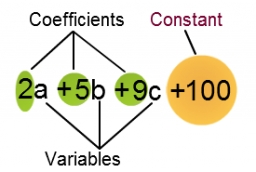Did you find an error or inaccuracy? Feel free to write us. Thank you!Tips to related online calculators
Need help to calculate sum, simplify or multiply fractions? Try our fraction calculator.

## Related math problems and questions:

• Fraction expressionWhich expression is equivalent to : minus 9 minus left parenthesis minus 4 start fraction 1 divided by 3 end fraction right parenthesis
• NumbersDetermine the number of all positive integers less than 4183444 if each is divisible by 29, 7, 17. What is its sum?
• Fraction unknowns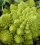Divide of fractions with unknowns: Fraction 1: The quantity x squared plus 6 times x plus 9 over the quantity x minus 1. Fraction 2: the quantity x squared minus 9 over the quantity x squared minus 2 times x plus 1.  Find Fraction 1 over Fraction 2.
• How many 15How many six-eights are there in 18? Which of the following would you choose to solve this question? 18 x 6/8 or 18 : 6/8
• Sum of fractionsWhat is the sum of 2/3+3/5?
• Multiplicative inverse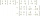Explain why the reciprocal of - 1 1/17 is the multiplicative inverse of - 17/18.
• Simplest form of a fractionWhich one of the following fraction after reducing in simplest form is not equal to 3/2? a) 15/20 b) 12/8 c) 27/18 d) 6/4
• RegroupingSubtract mixed number with regrouping: 11 17/20- 6 19/20
• Evaluate 12Evaluate and simplify 9 divided by 7/6.
• Factors 2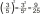A number has 3 identical factors. If 1 of them is 18. Find the number step by step
• Evaluate 17Evaluate 2x+6y when x=- 4/5 and y=1/3. Write your answer as a fraction or mixed number in simplest form.
• FractionFind for what x fraction (-4x -6)/(x) equals:
• Quotient and productIf the quotient of [8/5 divided by 8/10] is added to the product of [8/14 x 7/12 x 3/8], what is the sum?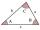The size of two internal angles of a triangle ABC are α=6/18π and β=7/18π. Calculate the size of the third angle.Evaluate expression: (-1)2 . 12 – 6 : 3 + (-3) . (-2) + 22 – (-3) . 2Add parenthesis to make true: 5-2×6-4+2=5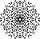What fraction of number 1 to 30 is prime?